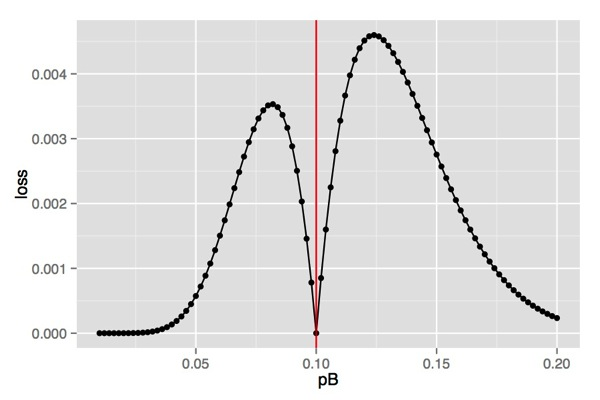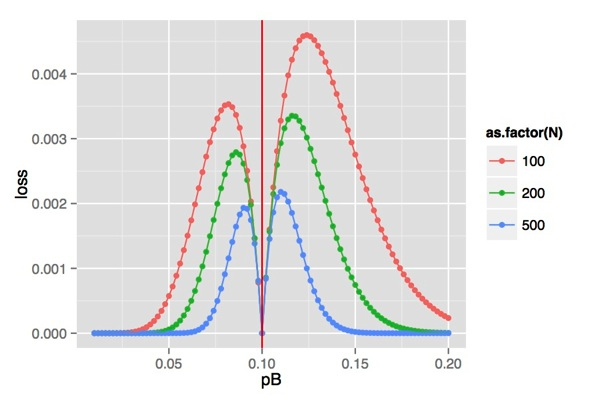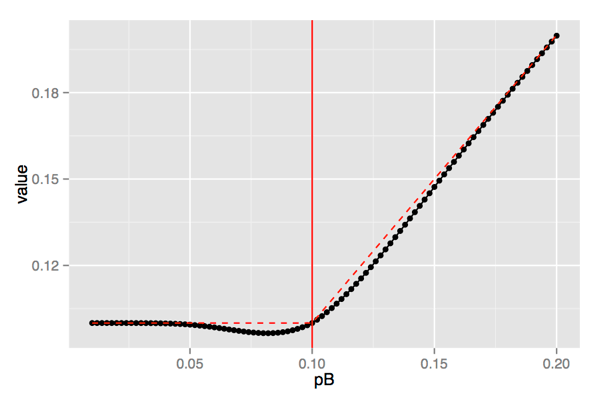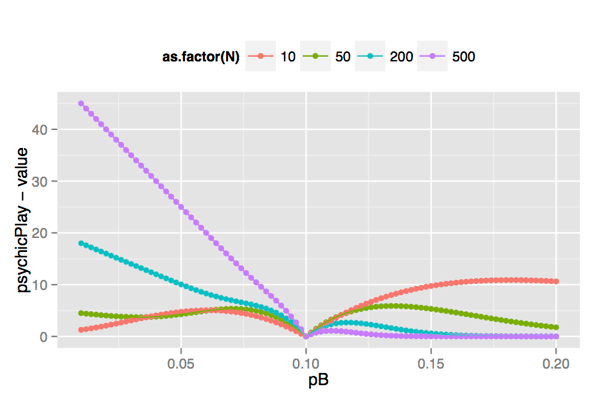# Bandit Formulations for A/B Tests: Some Intuition

Controlled experiments embody the best scientific design for establishing a causal relationship between changes and their influence on user-observable behavior.

— Kohavi, Henne, Sommerfeld, “Practical Guide to Controlled Experiments on the Web” (2007)

A/B tests are one of the simplest ways of running controlled experiments to evaluate the efficacy of a proposed improvement (a new medicine, compared to an old one; a promotional campaign; a change to a website). To run an A/B test, you split your population into a control group (let’s call them “A”) and a treatment group (“B”). The A group gets the “old” protocol, the B group gets the proposed improvement, and you collect data on the outcome that you are trying to achieve: the rate that patients are cured; the amount of money customers spend; the rate at which people who come to your website actually complete a transaction. In the traditional formulation of A/B tests, you measure the outcomes for the A and B groups, determine which is better (if either), and whether or not the difference observed is statistically significant. This leads to questions of test size: how big a population do you need to get reliably detect a difference to the desired statistical significance? And to answer that question, you need to know how big a difference (effect size) matters to you.

The irony is that to detect small differences accurately you need a larger population size, even though in many cases, if the difference is small, picking the wrong answer matters less. It can be easy to lose sight of that observation in the struggle to determine correct experiment sizes.

There is an alternative formulation for A/B tests that is especially suitable for online situations, and that explicitly takes the above observation into account: the so-called multi-armed bandit problem. Imagine that you are in a casino, faced with K slot machines (which used to be called “one-armed bandits” because they had a lever that you pulled to play (the “arm”) — and they pretty much rob you of all your money). Each of the slot machines pays off at a different (unknown) rate. You want to figure out which of the machines pays off at the highest rate, then switch to that one — but you don’t want to lose too much money to the suboptimal slot machines while doing so. What’s the best strategy?The “pulling one lever at a time” formulation isn’t a bad way of thinking about online transactions (as opposed to drug trials); you can imagine all your customers arriving at your site sequentially, and being sent to bandit A or bandit B according to some strategy. Note also, that if the best bandit and the second-best bandit have very similar payoff rates, then settling on the second best bandit, while not optimal, isn’t necessarily that bad a strategy. You lose winnings — but not much.

Traditionally, bandit games are infinitely long, so analysis of bandit strategies is asymptotic. The idea is that you test less as the game continues — but the testing stage can go on for a very long time (often interleaved with periods of pure exploitation, or playing the best bandit). This infinite-game assumption isn’t always tenable for A/B tests — for one thing, the world changes; for another, testing is not necessarily without cost. We’ll look at finite games below.

The Intuition

Let’s look at the simplest situation. We are in an existing situation A, with a known payoff rate, `pA`. We want to test a proposed improvement, B, with unknown payoff rate `pB`. Our testing strategy is to play the B situation `N` times, and at the end of that period, we will play whichever situation looks better. in other words, if our estimate of `pB` looks higher than `pA` at the end of the test period, then at the `N+1`th step we’ll play B; otherwise, we’ll go back to A. If we pick the right answer, we win. If you pick the wrong answer, then call `delta = abs(pA - pB)` the “opportunity loss”. What’s the expected opportunity loss on the `N+1`th turn? It’s delta times the probability of picking the wrong bandit.

If in reality `pB` is less than `pA`, then the probability of being wrong is the probability of flipping a coin with a `pB` heads-probability `N` times and seeing more than `ceiling(N*pA)` heads:

```pbinom(ceiling(N*pA), N, pB, lower.tail=F)
```

Or taking both situations (`pB <= pA and pB > pA)` into account:

```expectedLoss = function(pA, pB, N) {
delta = abs(pA - pB)

# The probability of seeing more than/less than pA*N heads in N flips,
# if the probability is really pB -- the probability of being wrong.
prob = (pB <= pA)*pbinom(ceiling(N*pA), N, pB, lower.tail=F) +
(pB > pA)*pbinom(ceiling(N*pA)-1, N, pB)

prob*delta
}
```

Let’s set `pA = 0.10` and `N=100`, and plot opportunity loss for different `pB`:

```library(ggplot2)

pA = 0.10
pBvec = seq(from = 0, to=0.2, by = 0.002)
loss100 = expectedLoss(pA, pBvec, 100)

ggplot(data.frame(pB=pBvec, loss=loss100), aes(x=pB, y=loss)) +
geom_point() + geom_line() + geom_vline(xintercept=pA, color="red")```As you can see in the figure, you don’t lose much when `pB` is much smaller or much larger than `pA`, because the probability of picking the wrong bandit is low. You don’t lose much when `pB` is very close to `pA`, because even if you pick the wrong bandit (fairly likely), the payoff rates are close. There is an intermediate difference (roughly 0.03 on either side of `pA`) where the difference in payoffs is notable, and the probability of picking the wrong bandit is fairly high, so the expected opportunity loss is maximized.

We can plot the loss curve for different values of `N` (same `pA`):As expected, the longer you test, the lower the expected loss on the next turn. The worst-case `pB` moves, too, and the region of largest loss gets smaller.

Of course, you might pick the right bandit, too. So the expected value of the next turn is:

```# after an N-length test, what's the expected value of a turn?
expectedValue = function(pA, pB, N) {

# case where pA => pB
areaBoverA = pbinom(ceiling(N*pA), N, pB, lower.tail=F)
# probability we guess wrong
value1 = (pB <= pA) * (areaBoverA*pB + (1-areaBoverA)*pA)
# case where pB > pA
areaAoverB = pbinom(ceiling(N*pA)-1, N, pB)
value2 = (pB > pA)*(areaAoverB*pA + (1-areaAoverB)*pB)

value1 + value2
}

# Expected value of a turn after 100 test-turns
val100 = expectedValue(pA, pBvec, 100)
ggplot(data.frame(pB=pBvec, value=val100), aes(x=pB, y=value)) +
geom_point() + geom_line() + geom_vline(xintercept=pA, color="red") +
geom_line(aes(y=pmax(pA, pB)), color="red", linetype=2)
```The above graph suggests that if you test `pB` for 100 turns, the expected value of the next turn goes to “the right answer” for `pB` outside the region `(0.05, 0.18)`. If you test `pB` longer, you can even shrink that region. If your game is infinitely long (that is, you will go with your chosen bandit from turn `N+1` on, forevermore), then whatever opportunity you lost during the testing phase is a negligible part of your total expected value, and it’s in your interest to test for a very long time (this is not, however, the best way to play an infinite-length bandit game).

Finite Games

But games aren’t necessarily infinite, as we mentioned above. Let’s look at a simple finite game. As before, `pA` is known, `pB` is what you want to test. The entire game consists of `M` turns, and you will spend the first `N` turns testing `pB`. After that, you play the bandit that appears to have the higher payoff rate for the remainder of the game (we’ll call that the exploitation phase). Now what’s the best choice of `N`?

The expected value of the entire game is the value of the testing phase, `pB*N`, plus the expected value of the rest of the game: `expectedValue(pA, pB, N)*(M-N)`. We can compare that to the perfect game, where you psychically know which bandit is better, and play that one for the entire game: `pmax(pA, pB)*M`.

```gameMatrix = function(pa, pb, M, N) {
psychicPlay = pmax(pa,pb)*M
valueTest = pb*N
expValuePlay = expectedValue(pa, pb, N)*(M-N)
data.frame(pB=pb, testValue=valueTest, expPlayVal=expValuePlay,
value=valueTest+expValuePlay,psychicPlay=psychicPlay,N=N)
}```

We can evaluate a 1000-turn game for different values on `N` (`pA = 0.1`), and plot the expected total opportunity loss, as compared to the perfect game:If `pB > pA`, then it’s best to play for a long time; you are not losing opportunity during the test phase, and you will only lose opportunity in the exploitation phase if you incorrectly choose `pA` — so test long enough to make that unlikely. If `pB < pA`, you are losing opportunity in the testing phase, which argues for a small `N`, but if you don’t test long enough, you are more likely to pick the wrong bandit at the end of the test phase — and hence will lose even more opportunity. On the other hand, if `pB` is very small, you are “wasting” opportunity by continuing to test even after it’s clear that `pB < pA`, and so losing opportunity when you could be playing optimally (that’s why the loss curve dips up again as `pB` approaches zero). The trick is to balance the tradeoffs.

Imagine that the universe is actively working against you: no matter what `N` you choose, the universe arranges that bandit B will have the worst possible `pB` for that testing length. Then, for all the test lengths that you want to consider, figure out what that worst-case `pB` would be, and its expected opportunity loss. Call that `maxloss_N`. The best N to use is the N for which `maxloss_N` is minimized.

```N = seq(from=10, to=200, by=10)
for(n in N) {
if(n==10) {gameValue = gameMatrix(pA, pBvec,M, n)}
else {gameValue = rbind(gameValue, gameMatrix(pA, pBvec, M, n))}
}

#
# I'm using sqldf to do the "group by", but you can use
# aggregate() or a similar function instead.
#
options(gsubfn.engine="R") # need this on a Mac
library(sqldf)
maxloss = sqldf('select N, max(psychicPlay-value) as mloss
from gameValue
group by N')

```For the game we are playing, `N = 50` is the best choice. The maximum expected loss occurs at `pB = 0.134`, with an expected value of 128.1; that’s a loss of 5.89 payoffs, or 4.4% fewer than the perfect game for that value of `pB`, which has an expected value of 134 (1000*0.134). There’s another local maxima at `pB = 0.072`, with an expected value of 94.65. Compared to the perfect game’s expected value of 100, that’s a loss of 5.35 payoffs, or 5.35% of the perfect game.

Compare this to the number of times you would have to play bandit B at `pB = 0.134` or `pB = 0.07` in order to separate it from bandit A to p = 0.05 significance:

```library(gtools)

tailprobs = function(pA, pB, N) {
if(pB <= pA) {
prob = pbinom(ceiling(N*pA), N, pB, lower.tail=F)
else { #(pB > pA)
prob = pbinom(ceiling(N*pA)-1, N, pB)
}
prob
}

#
# Do binary search to find the minimum N that achieves
# the desired significance
#

sigtarget = 0.05
s1 = binsearch(function(k) {tailprobs(pA, 0.134, k) - sigtarget},
range=c(1,ceiling(10000/pA)))
max(s1\$where)
# 256

s2 = binsearch(function(k) {tailprobs(pA, 0.07, k) - sigtarget},
range=c(10,ceiling(100/pA)))
max(s2\$where)
# 131
```

Given the numbers above, we know that if `pB` is about 0.03 away from our `pA`, an `N=50` game with the given parameters will make a lot of mistakes identifying which bandit has the better payoff — but we also know from our previous analysis that (assuming we don’t know the true `pB`) the lost opportunity costs are as low as we can make them. Unless it is absolutely critical that you identify the correct bandit, the above analysis shows that it possible to achieve utility before you achieve significance.

Tagged as:### Nina Zumel

Data scientist with Win Vector LLC. I also dance, read ghost stories and folklore, and sometimes blog about it all.

### 2 replies ›

1.David Gonzales says:

hello,

in the function, expectedLoss you use the expression:
pbinom(ceiling(N*pA)-1

the -1 is perhaps superfluous as the lower.tail=F and the normal pbinom add to 1.0 (as the total probability).
perhaps I am somehow confused.

1.Nina Zumel says:

I want to make both intervals (left tail and right tail) exclusive, as I am looking at the cases where pB is strictly greater or strictly less than pA. That said, the end point calculations can be a bit tricky, and the -1 may be unneeded. I will recheck. Thanks!